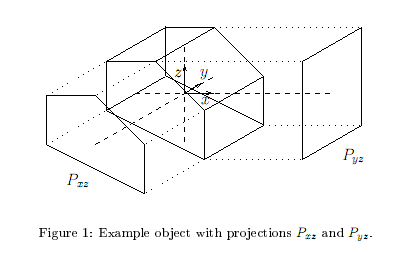## Дата и время

07/12/2023 22:05:57

## Авторизация

 Имя: Пароль:
Зарегистрироваться
Восстановить пароль

#Тренировка 4

##A. Geodes

Ограничения: время – 2s/4s, память – 64MiB Ввод: input.txt или стандартный ввод Вывод: output.txt или стандартный выводПослать решение Blockly Посылки Темы Где Обсудить (0)

In the mountains of Geodesia, geodes are found. These 'hollow' stones contain cavities with crystal formations around them. The beautifully colored crystals can be sold for a high price to people both inside and outside of Geodesia. The problem with geodes is that one cannot see from the outside of a given rock whether it is a geode or not. Out of every thousand rocks found in Geodesia only some are geodes.
In order to find out whether a given rock is in fact a geode, one can try to consider its density
ρ\ =\ m/V,
where m represents its mass and V its volume. Since a geode contains empty space, a rock contaning one is expected to have a lower density than other rocks. If the density of a rock is too high, it would be a waste of time and effort to further investigate it.
But how could one determine the density of each of the enormous number of rocks found in the mountains of Geodesia? Minig crafts collect rocks, which they put on mobile conveyer belts. Weighing the rocks automatically at a fast rate is no problem, but how to quickly determine their volume? Measuring e.g. the volume displacement of a liquid(like H_2\ O), where each rock has to be put in and taken out individually is a time consuming process.
So why not try to estimate the volume just by looking at the rock? Along the conveyer belt, two cameras are placed, perpendicular to each other. Of every rock that passes, they each take a picture. These pictures are sent to a computer for processing. The computer calculates from these pictures an upper bound for the volume of the rock, V_max. A lower bound for the density of the rock is then given by
ρ_min\ =\ m/V_max\ ≤\ m/V\ =\ ρ.
This lower bound can then be used to reject rocks that are certainly too heavy to be geodes.
The computer uses a relatively simple trick for estimating the maximal volume. It considers the pictures taken as silhouettes, as parallel projections of a rock onto a planes. Since the two cameras are placed perpendicular to each other, the projection planes are also perpendicular to each other.
In other words, choose a coordinate system in three dimensional space as follows: z runs along the vertical direction and x and y run along the perpendicular horizontal directions in which the two cameras are placed. Let S\ ⊂\ R^3 represent a rock. Then the cameras yield the two parallel projections
P_"xz"\ =\ {\ (x,z)\ :\ (x,y,z)\ ∈\ S\ ∀\ y\ ∈\ R\ } and
P_"yz"\ =\ {\ (y,z)\ :\ (x,y,z)\ ∈\ S\ ∀\ x\ ∈\ R\ }
where "xz" and "yz" plane respectively. See Figure 1 for an example.Now a curious property of the rocks found in Geodesia makes the measurement of the volume easier: they are all convex1. Furthermore, the projections can be considered as polygons. So first each of the two pictures taken of a rock is converted into a convex polygon by an image recognition program. You are to write a program that will do the second step: compute from these two polygons V_max: the maximum volume of any rock having exactly these two polygon-shaped projections.
Input
The first line of input contains a single number: the number of test cases to follow. Each test case has the following format:
One line with the integer n_"xz" the number of vertices describing the projection on the "xz" plane.
n_"xz" lines, each with two integers x and z, separated by a single space: the coordinates of one vertex of the "xz" projection.
One line with the integer n_"yz", the number of vertices describing the projection on the "yz" plane.
n_"yz" lines, each with two integers y and z, seperated by a single space: the coordinates of one vertex of the yz projection.
Both projections are convex polygons, and the minimum and maximum z coordinates are the same values. The vertices of the polygons are given in clockwise order. Consecutive vertices of the polygons are different, but there may be three or more consecutive vertices on one line. The numbers in the input satisfy 3\ ≤\ n_"xz",\ n_"yz"\ ≤\ 1000, and |x|,\ |y|,\ |z|\ ≤\ 500.
Output
For every test case in the input, the output should contain a single number on a single line: V_max, the maximum volume of any object having the projections given in the input, rounded in the usual way to two decimals behind the decimal point, A round-off error of 0.01 is permitted in your answer.

Sample Input (figure 1)

1
5
1 0
1 -1
-1 0
-1 1
0 1
5
-1 1
0 1
1 1
1 -1
-1 -1


Sample Output

5.00

Source: Benelux Algorithm Programming Contest 2006